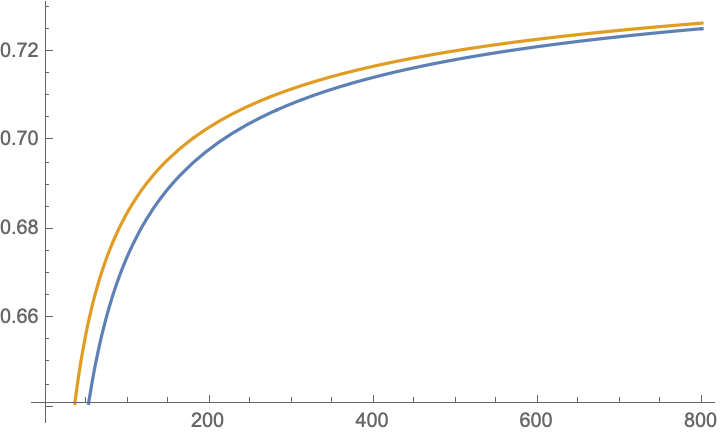# Help with a limit involving incomplete beta integral

In trying to prove that the limit of a certain function approaches 1 as the positive integer parameter $$n$$ approaches infinity, I have ended up with the following intermediate expressions: $$f(n)=2^{1+2n}B_{1/2}(n,n+2)$$ $$g(n)=4^nB_{1/2}(n+1,n)$$ $$h(n)=n(n-1)/2 \left( \frac{f(n)}{n+1}-\frac{g(n)}{n-1}\right)$$ Can somebody kindly help me with the evaluation of $$\lim_{n \to \infty} h(n)$$?
If somebody could also plug it in Mathematica, I would be highly obliged. Thanks for any help in advance.
P.S.: In the above the notation $$B_z(a,b)$$ stands for the incomplete beta function defined by: $$B_z(a,b)=\int\limits_0^z u^{a-1}(1-u)^{b-1} \mathrm{d}u.$$

• $z \to B_z(a,b)$ is not the incomplete beta function. You have to divide by $B(a,b) := B_1(a,b)$. $B(a,b)$ can be simply computed as a quotient of $\Gamma$-functions. If I remember correctly its just $\frac{\Gamma(a+b)}{\Gamma(a)\Gamma(b)}$. Mar 5, 2021 at 10:44
• @DieterKadelka --- the formula in the OP uses the definition of the incomplete beta function on en.wikipedia.org/wiki/Beta_function#Incomplete_beta_function ; what you write is called the "regularized" incomplete beta function on Wikipedia. Mar 5, 2021 at 10:57

Using the substitution $$u=(1-s)/2$$ in the relevant integrals $$\int_0^{1/2} u^{a-1}(1-u)^{b-1} \,du$$, then using the substitution $$s^2=t$$, and finally noting that $$\Gamma(x+1/2)/\Gamma(x)\sim\sqrt x$$ as $$x\to\infty$$, we have $$h(n)=\frac n{2(n+1)}\,\int_0^1 ds\,(1 - s^2)^{n - 1} ((n - 1) s^2 + (3 n - 1) s - 2) \\ =\frac n{8(n+1)}\,{\left(6-\frac2n-\frac{3 \sqrt{\pi }\, \Gamma (n+2)}{n\Gamma \left(n+3/2\right)}\right)}\to\frac68$$ as $$n\to\infty$$.

• that cannot be quite correct, this expression diverges as $-\sqrt n$ for large $n$; I think your factor $\Gamma(n+2)$ should be $n\Gamma(n)$ like in my surmise. Mar 5, 2021 at 16:08
• @CarloBeenakker : Thank you for your comment. There was a typo: the factor $n$ in the denominator $n\Gamma\left(n+3/2\right)$ was missing. this is now fixed. Mar 5, 2021 at 16:18
• I meant the $h(n)=\frac{n}{4(n+1)}[\frac{(n-1)\Gamma(n)\sqrt{\pi}}{2\Gamma(n+3/2)}+3-\frac{1}{n}-\frac{2\Gamma(n)\sqrt{\pi}}{\Gamma(n+1/2)}]$. Probably it's same as the identity in the answer now. By the way, nice answer. Mar 5, 2021 at 16:36
• @AlapanDas : Thank you for your comment. Yes, your expression for $h(n)$ is the same as the one in this answer. Mar 5, 2021 at 16:55

The blue line is the numerical evaluation of $$h(n)$$, the gold line is

$$H(n)=\frac{3}{4}-\frac{3 \sqrt{\pi } n \Gamma (n)}{8 \Gamma \left(n+\frac{3}{2}\right)}\rightarrow \frac{3}{4}$$• Mathematica can compute the limit as well .u may kindly copy f[n] = 2^(1 + 2 n) Beta[1/2, n, 2 + n] g[n] = 4^n Beta[1/2, 1 + n, n] f1[n] = f[n]/(n + 1) g1[n] = g[n]/(n - 1) l[n] = n (n - 1)/2 (f1[n] - g1[n]) Limit[l[n], n -> Infinity] Mar 5, 2021 at 8:20
• that does not work, but I made an "educated guess", which seems to agree quite well numerically. Mar 5, 2021 at 10:05

Python gives values upto $$n=1000$$. Values of $$h(n)$$ for $$997 \leq n \leq 1000$$ are $$f(997)= 0.728174227325497 ,f(998)=0.7281857363386501 ,f(999)= 0.7281972275318511 ,f(1000)=0.7282087009506559$$. Also, $$f(900)=0.7269653136612485$$The Code:

import matplotlib.pyplot as plt
import numpy as np
import math as m

def f(x):
f=0
g=0
h=0.001
for i in range(0,2000):
u=i*h
f=f+2*(u**(x-1))*((1-u/4)**(x+1))*h*(x-1)
g=g+0.25*(u**x)*((1-u/4)**(x-1))*h*(x+1)

v=x/(x+1)*(f-g)/2
return v

x=np.linspace(2, 1000, 1000)
F=np.vectorize(f)
plt.plot(x,F(x),'r')
plt.xlabel('n')
plt.ylabel('h(n)')
plt.show()

• ,kindly share the python program as i am not able to access the file in colab because of permission issues Mar 5, 2021 at 9:54
• scipy.sci.btdtr(a,b,x) in the module scipy computes the incomplete $B_x(a,b)$, I doubt if this is of any use for the limit due to floating point limits. Mar 5, 2021 at 10:07
• @Dieter Kadelka thank you. I shall use this function. Mar 5, 2021 at 10:09